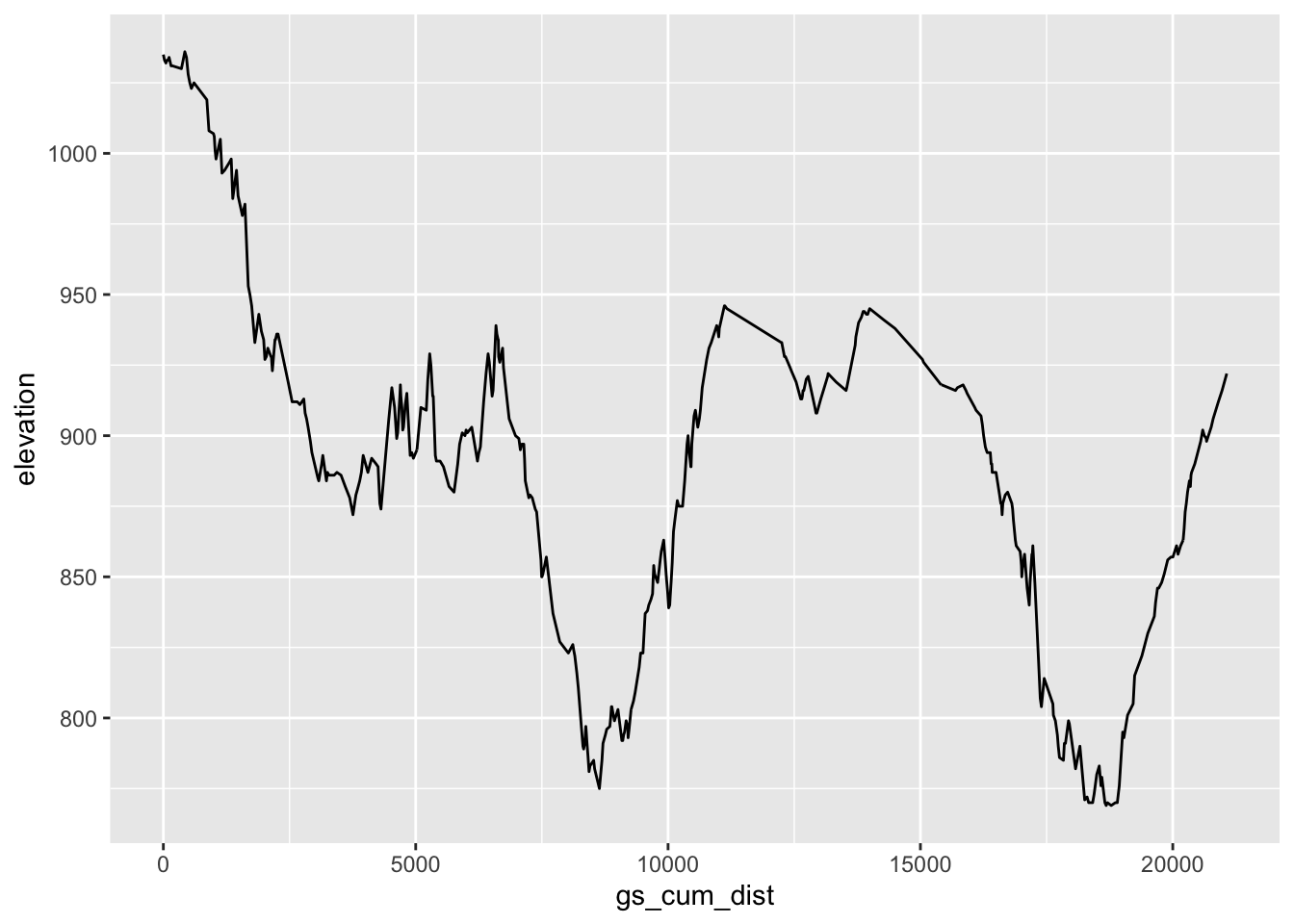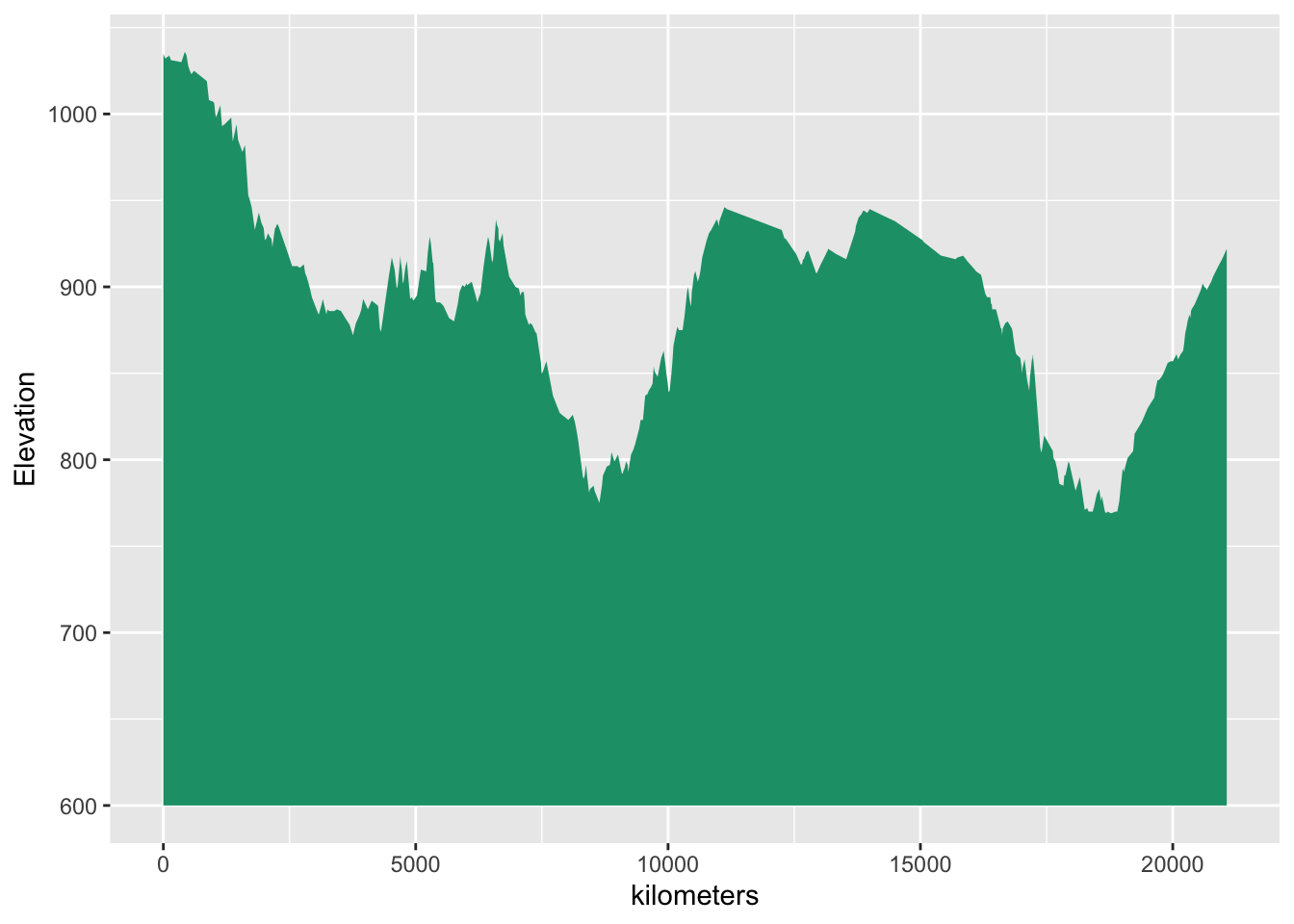# 18 Elevation Data Revisited

Having access to elevation data is essential for rendering 3D terrain maps. But we can also plot elevation data along a single dimension: the distance along route.

For winter mountain rallies, such as Monte Carlo, elevation data may be important when estimating temperature drops along a stage caused by elevation changes, and hence the likelihood, or otherwise, of snow. (Shadow models and the time of day a stage is running, as well as the weather conditions, may also play into that).

When planning hill climbs, or rally stages that perhaps involve electric vehicle competitors, a good understanding of elevation changes, and potential energy requirements resulting from climbs, may also be important,

Rally Maps routes come with elevation profiles of this sort, and stage reports for major cycling races such as le Tour de France are rarely complete with a stage elevation maps show the cols to be encountered along the route. The `cyclingcols.com` website demonstrates several interesting ways of analysing cycling cols.

So can we produce our own elevation profiles from the stage route and elevation data? We surely can…

Elevation along route profiles are extracted from an elevation raster. Note that we can also extract shadow along route information from a shade raster generated for a particular data and time of day.

## 18.1 Load in Base Data

As ever, let’s load in our stage data and the elevation raster and create a demo map:

``````library(sf)
library(raster)

geojson_filename = 'montecarlo_2021.geojson'
``````## Reading layer `montecarlo_2021' from data source `/Users/tonyhirst/Documents/GitHub/visualising-rally-stages/montecarlo_2021.geojson' using driver `GeoJSON'
## Simple feature collection with 9 features and 2 fields
## geometry type:  LINESTRING
## dimension:      XY
## bbox:           xmin: 5.243488 ymin: 43.87633 xmax: 6.951953 ymax: 44.81973
## geographic CRS: WGS 84``````
``````stage_route_gj = geojsonio::geojson_json(geojson_sf[1,]\$geometry)

stage_tif = "buffered_stage_elevation.tif"

# Load in the previously saved image raster
elev_img = raster(stage_tif)

# Note we can pass in a file name or a raster object
elmat = raster_to_matrix(stage_tif)``````
``##  "Dimensions of matrix are: 468x626."``
``````demo_map = elmat %>%
sphere_shade(texture = "desert", progbar = FALSE)``````

## 18.2 Generating Elevation Maps

With the geo data provided for the stage route, we only have access to the latitude and longitude of each point along the route, not the elevation.

We have already seen how we can create a route layer that we can overlay on the 3D model, with the elevation then inferred so that the route is plotted correctly on the 3D rendered model. But can we access the actual elevation values along the route somehow?

### 18.2.1 Accessing Elevation Values Along a Route from an Elevation Raster

The elevation raster provides an elevation value for each pixel in the raster image. We can transect the raster with a route to get the elevation value for each point along the route.

To get a routepoints list, we can extract the coordinates from a linestring geometry, casting the result to a dataframe and then selecting just the X (longitude) and Y (latitude) values:

``````routepoints = subset(as.data.frame(st_coordinates(geojson_sf[1,])),
select = c('X', 'Y'))
``````##          X        Y
## 1 5.894486 44.73562
## 2 5.894618 44.73580
## 3 5.894778 44.73602
## 4 5.894805 44.73621
## 5 5.894672 44.73636
## 6 5.894407 44.73642``````

We can add a Z (elevation) column to the dataframe by extracting the elevation at each point (that is, for each row of the dataframe) from the elevation raster image:

``````routepoints\$elevation = raster::extract(elev_img, routepoints)
``````##          X        Y elevation
## 1 5.894486 44.73562      1035
## 2 5.894618 44.73580      1033
## 3 5.894778 44.73602      1032
## 4 5.894805 44.73621      1033
## 5 5.894672 44.73636      1033
## 6 5.894407 44.73642      1034``````

Finding the minimum and and maximum altitude along the route is trivial:

``c(max(routepoints\$elevation, na.rm=T), min(routepoints\$elevation, na.rm=T))``
``##  1036  769``

### 18.2.2 Using `geosphere::distGeo` to Find Distance Along a Route

We can use the `geosphere::distGeo()` function to calculate distance in meters between each point under the WGS84 projection without having to first convert to a projection with units of meters.

The function returns the distance between consecutive locations, with a null value returned for the final step. If we want to back refer distances — how far since the last point, rather than how far to the next point — we can drop the last `NA` distance and prepend a distance of `0` to the start of the list of distances:

``````stage_coords = st_coordinates(geojson_sf[1,])[,c('X','Y')]
stage_step_distances = geosphere::distGeo(stage_coords)

# The last step distance is NA, so drop it
# Also prepend the distances with 0 for the first step
routepoints\$gs_cum_dist = cumsum(routepoints\$gs_dist)

``````##          X        Y elevation  gs_dist gs_cum_dist
## 1 5.894486 44.73562      1035  0.00000     0.00000
## 2 5.894618 44.73580      1033 22.57054    22.57054
## 3 5.894778 44.73602      1032 27.93282    50.50337
## 4 5.894805 44.73621      1033 21.00098    71.50435
## 5 5.894672 44.73636      1033 20.38067    91.88502
## 6 5.894407 44.73642      1034 21.77090   113.65592``````

We can now preview the elevation against the distance along the route:

``````library(ggplot2)

ggplot(routepoints, aes(x=gs_cum_dist, y=elevation)) + geom_line()``````### 18.2.3 Using `sp::spDists` to Find Distance Along a Route

The `sp::spDists` function also allows us to find the distance between Spatial point WPS84 / longlat projected points in meters without first having to convert the points to a projection with units of meters. The function returns the “step” distance as well as the accumulated distance:

``````# Find the distance between points in two lists of coordinates
distances = sp::spDists(rbind(stage_coords[1,], stage_coords),
stage_coords,
longlat = TRUE)

# Extract the step and accumulated distances in km
# and convert to meters
routepoints['sp_cum_dist'] = distances[1,] * 1000
routepoints['sp_dist'] = distances[2,] * 1000

``````##          X        Y elevation  gs_dist gs_cum_dist sp_cum_dist  sp_dist
## 1 5.894486 44.73562      1035  0.00000     0.00000     0.00000  0.00000
## 2 5.894618 44.73580      1033 22.57054    22.57054    22.57069 22.57069
## 3 5.894778 44.73602      1032 27.93282    50.50337    50.50298 50.50298
## 4 5.894805 44.73621      1033 21.00098    71.50435    70.47300 70.47300
## 5 5.894672 44.73636      1033 20.38067    91.88502    84.52844 84.52844
## 6 5.894407 44.73642      1034 21.77090   113.65592    89.23297 89.23297``````

Note that the actual distances calculated vary slightly depending on the internals and settings used in the function used to calculate them. The numbers are close enough for storytelling!

## 18.3 Generating a 3D Ribbon Plot of the Stage Route

A `ggplot2::geom_ribbon()` plot provides a familiar way of rendering the elevation plot in the form a line chart with a fill that extends down to the x-axis:

``````library(ggplot2)

# Find a nice minimum elevation
min_elevation = max(0, 100*round(((min(routepoints\$elevation)/100)-1.6)))
max_elevation = max(routepoints\$elevation)

g = ggplot(routepoints, aes(x = gs_cum_dist)) +
geom_ribbon(aes(ymin = min_elevation,
ymax = elevation),
fill = "#1B9E77") +
labs(x = "kilometers", y = "Elevation") +
scale_y_continuous(limits = c(min_elevation, max_elevation))

g``````We can get a 3D plot from the ribbon chart via the `rayshader::plot_gg()` function:

``````options(rgl.useNULL = TRUE,
rgl.printRglwidget = TRUE)

rgl::clear3d()
plot_gg(g, height=5, width=6, scale=500, raytrace=FALSE)

rgl::rglwidget()``````